# Can Ravens Forecast?

R
time series
forecast
Time series forecasting using cloud services spend data
Author

Carl Goodwin

Published

July 29, 2018

Modified

January 21, 2023Humans have the magical ability to plan for future events, for future gain. It’s not quite a uniquely human trait. Because apparently ravens can match a four-year-old.

An abundance of data, and some very nice R packages, make our ability to plan all the more powerful.

In the Spring of 2018 I looked at sales from an historical perspective in Six Months Later.. Here I’ll use the data to model a time-series forecast for the year ahead. The techniques apply to any time series with characteristics of trend, seasonality or longer-term cycles.

Why forecast sales? Business plans require a budget, e.g. for resources, marketing and office space. A good projection of revenue provides the foundation for the budget. And, for an established business, with historical data, time-series forecasting is one way to deliver a robust projection.

Without exogenous data, the forecast assumes one continues to do what one’s doing. So, it provides a good starting-point. Then one might, for example, add assumptions about new products or services. And, if there is forward-looking data available, for example, market size projections (with past projections to train the model), then one could feed this into the forecast modelling too.

``````library(conflicted)
library(tidyverse)
conflict_prefer_all("dplyr")
library(wesanderson)
library(fpp3)
library(scales)
library(clock)
library(usedthese)

conflict_scout()``````
``````7 conflicts:
* `as_date`    : clock, lubridate
* `date_format`: clock, scales
* `filter`     : [dplyr]
* `interval`   : tsibble, lubridate
* `lag`        : [dplyr]``````
``````theme_set(theme_bw())

(cols <- wes_palette(name = "IsleofDogs2"))``````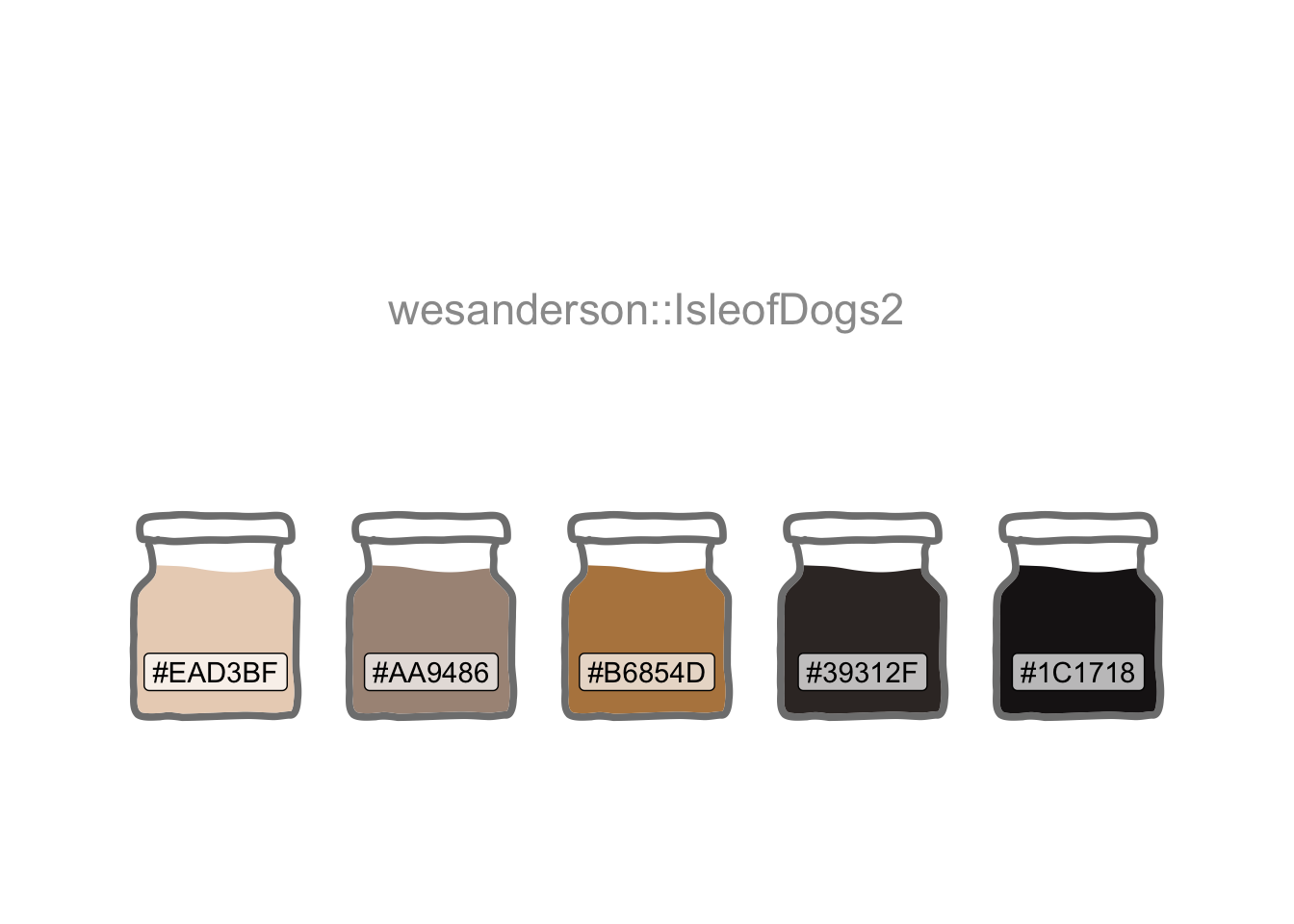First I’ll check the encoding of the data.

``````url <-

gcloud_csv <- str_c(url, "703943/G-Cloud_spend_data_to_end_March_2018.csv")

dos_csv <- str_c(url, "703952/DOS_spend_data_to_end_March_2018.csv")

names <- c(gcloud_csv, dos_csv)

# Use walk to suppress the printing of list element numbers

walk(names, \(x) {
p <- guess_encoding(x)
print(p)
})``````
``````# A tibble: 2 × 2
encoding   confidence
<chr>           <dbl>
1 ISO-8859-1       0.4
2 ISO-8859-2       0.22
# A tibble: 2 × 2
encoding   confidence
<chr>           <dbl>
1 ISO-8859-1       0.36
2 ISO-8859-2       0.24``````

Next I’ll set up a vector of column names to apply consistently to both files, and import the data with the suggested encoding.

``````colnam <-
c("sector",
"lot",
"date",
"spend",
"status",
"supplier",
"customer",
"framework")

x,
col_names = colnam,
skip = 1,
locale = locale(encoding = "ISO-8859-1"),
show_col_types = FALSE)
}

set_names(c("gcloud", "dos")) |>
bind_rows() |>
mutate(framework = if_else(is.na(framework), "DOS", framework))``````

I’d like to create some new features: Month-end dates, something to distinguish between the two frameworks (G-Cloud or DOS). The spend has a messy format and needs a bit of cleaning too.

The lot structure for G-Cloud has evolved over time, but fortunately, there is a simple mapping, i.e. PaaS and IaaS became Cloud Hosting, SaaS became Cloud Software, and Specialist Cloud Services became Cloud Support, so I’ll standardise on the latter.

``````both <- raw |>
mutate(
month_end = date_parse(str_c(date, "01", sep = "-"),
format = "%b-%y-%d") |>
date = yearmonth(month_end),
framework = str_extract(framework, ".{3,7}"),
spend = str_remove(spend, coll("£")),
spend = str_replace(spend, "^\\(", "-"),
spend = parse_number(spend) / 1000000,
lot = recode(
lot,
"Software as a Service (SaaS)" = "Cloud Software",
"Infrastructure as a Service (IaaS)" = "Cloud Hosting",
"Platform as a Service (PaaS)" = "Cloud Hosting",
"Specialist Cloud Services" = "Cloud Support"
)
)``````

The tidied data now needs to be converted to a tsibble, the temporal equivalent of a tibble.

R has evolved since I first wrote this post. At that time, it was necessary to either split the data into the two frameworks (G-Cloud and DOS) and forecast them separately. Or, as I did with the three G-Cloud lots, use the purrr package to iterate through a forecast.

The tsibble package combined with the newer fable and feasts packages, make this easier. One of the defining feature of the tsibble is the `key`. I want a model for each framework, so I’m setting this as the tsibble `key` (and the temporal variable as the tsibble `index`).

``````both_ts <- both |>
summarise(spend = sum(spend), .by = c(date, framework)) |>
as_tsibble(key = framework, index = date)

both_ts |>
ggplot(aes(date, spend, colour = framework)) +
geom_line(key_glyph = "timeseries") +
scale_y_continuous(labels = label_dollar(prefix = "£", suffix = "m")) +
scale_colour_manual(values = cols[c(3, 4)]) +
labs(x = NULL, y = NULL, title = "Monthly Digital Marketplace Sales")``````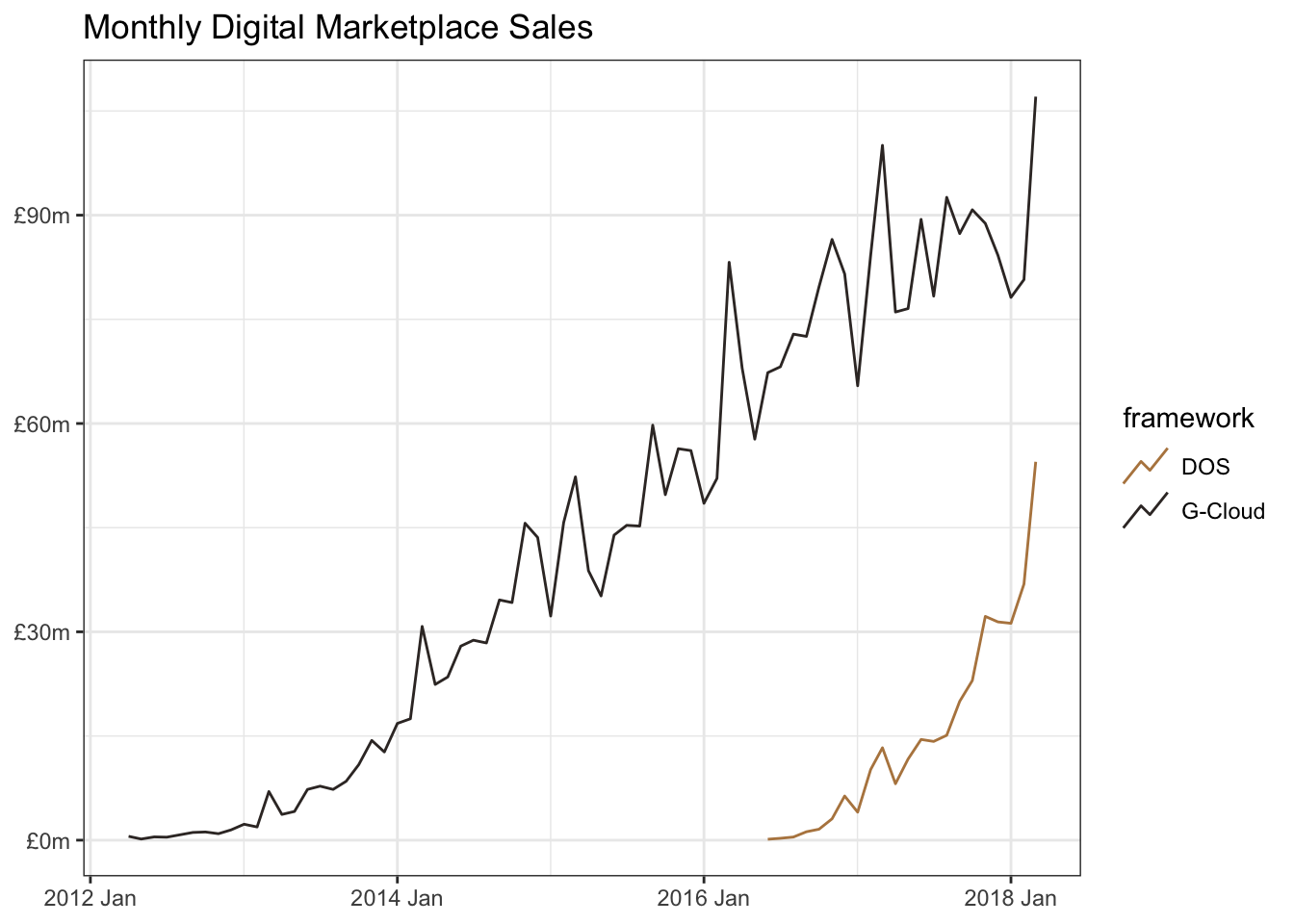By decomposing the historical data we can tease out the underlying trend and seasonality:

• Trend: The sales for both frameworks have grown over time as more Suppliers have added their services to the Government frameworks, and more Public Sector organizations have found the benefits of purchasing Cloud services through this faster, simpler, more transparent and more competitive contracting vehicle.

• Seasonality: Suppliers often manage their sales and financials based on a quarterly cycle, with a particular emphasis on a strong close to the financial year. And Government Buyers may want to make optimal use of their budgets at the close of their financial year (March 31st). Consequently, we see quarterly seasonality with an extra spike at financial year-end.

``````both_ts |>
model(stl = STL(spend ~ trend(window = 7) + season(window = "periodic"))) |>
components() |>
autoplot() +
scale_colour_manual(values = cols[c(3, 4)]) +
labs(x = NULL, title = "Time Series Decomposition")``````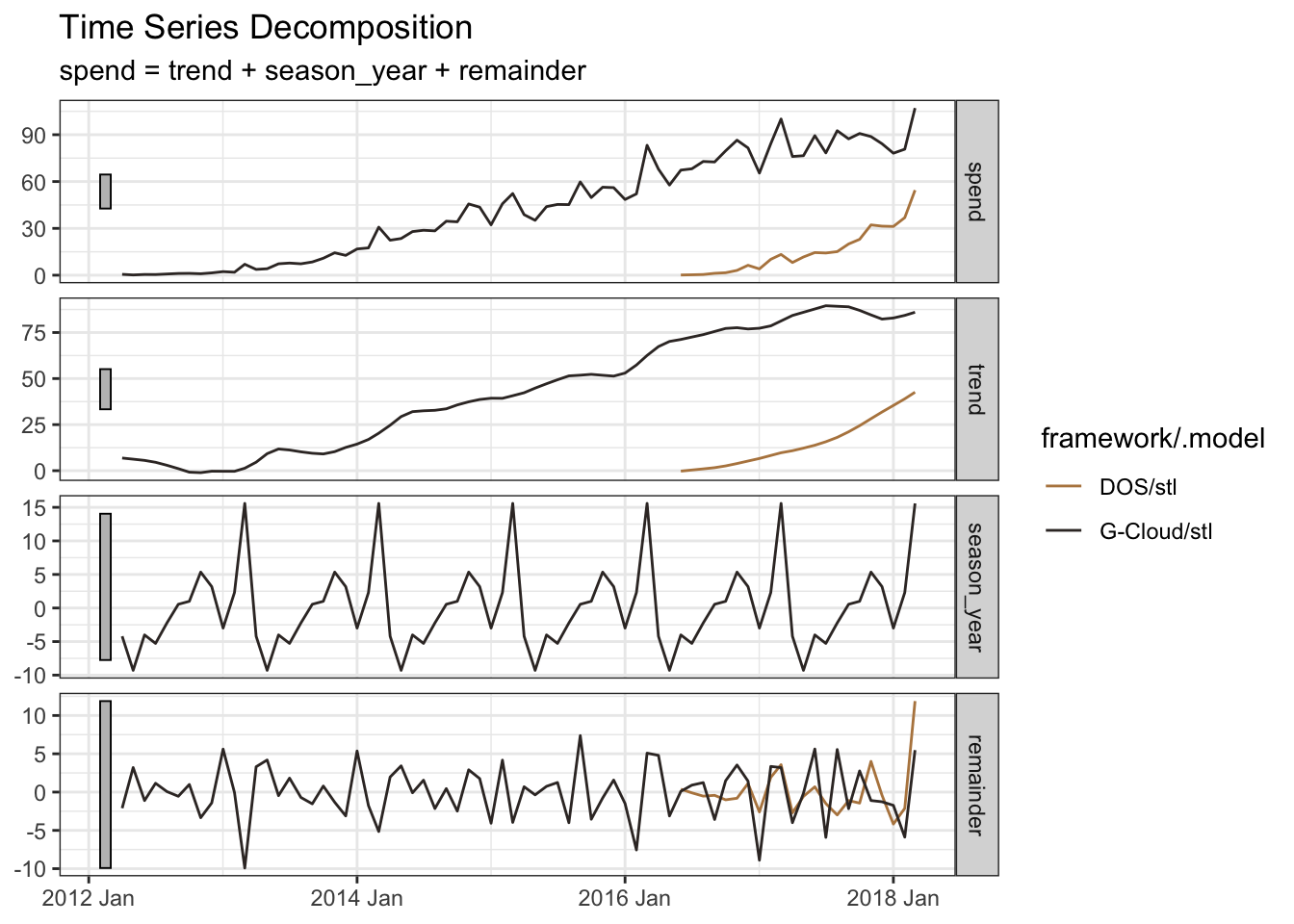I’ll use `auto.arima`: AutoRegressive Integrated Moving Average modelling which aims to describe the autocorrelations in the data.

By setting `stepwise` and `approximation` to `FALSE`, `auto.arima` will explore a wider range of potential models.

I’ll forecast with the default 80% and 95% prediction intervals. This means the darker-shaded 80% range should include the future sales value with an 80% probability. Likewise with a 95% probability when adding the wider and lighter-shaded area.

Use of `autoplot` would simplify the code, but personally I like to expose all the data, for example unpacking the prediction intervals, and have finer control over the visualisation.

``````mod_ts <- both_ts |>
model(ARIMA = ARIMA(spend, stepwise = TRUE, approximation = FALSE))

mod_ts |>
glance() |>
select(-ar_roots, -ma_roots)``````
framework .model sigma2 log_lik AIC AICc BIC
DOS ARIMA 23.60154 -59.93305 123.8661 124.5720 125.8576
G-Cloud ARIMA 34.75275 -191.61547 393.2309 394.3421 403.7027
``````mod_ts |>
tidy()``````
framework .model term estimate std.error statistic p.value
DOS ARIMA ma1 -0.7725018 0.1718541 -4.495102 0.0002213
G-Cloud ARIMA ar1 0.9390150 0.0595354 15.772391 0.0000000
G-Cloud ARIMA ma1 -0.5777210 0.1094066 -5.280494 0.0000019
G-Cloud ARIMA sar1 -0.5124417 0.1142670 -4.484597 0.0000336
G-Cloud ARIMA constant 1.4084703 0.3168155 4.445712 0.0000385
``````fcast_ts <- mod_ts |>
forecast(h = "2 years") |>
mutate(`95%` = hilo(spend, 95), `80%` = hilo(spend, 80)) |>
unpack_hilo(c("95%", "80%")) |>
rename(fc_spend = spend) |>
bind_rows(both_ts)

fcast_ts |>
ggplot(aes(date, fill = framework)) +
geom_line(aes(y = spend), colour = cols) +
geom_ribbon(aes(ymin = `95%_lower`, ymax = `95%_upper`),
fill = cols, colour = NA
) +
geom_ribbon(aes(ymin = `80%_lower`, ymax = `80%_upper`),
fill = cols, colour = NA
) +
geom_line(aes(y = .mean), colour = "white") +
scale_y_continuous(labels = label_dollar(prefix = "£", suffix = "m")) +
facet_wrap(~framework) +
labs(
title = "Digital Marketplace Sales Forecast by Framework",
x = NULL, y = "Spend",
subtitle = "80 & 95% Prediction Intervals"
) +
theme(
legend.position = "none",
axis.text.x = element_text(angle = 45, hjust = 1)
)``````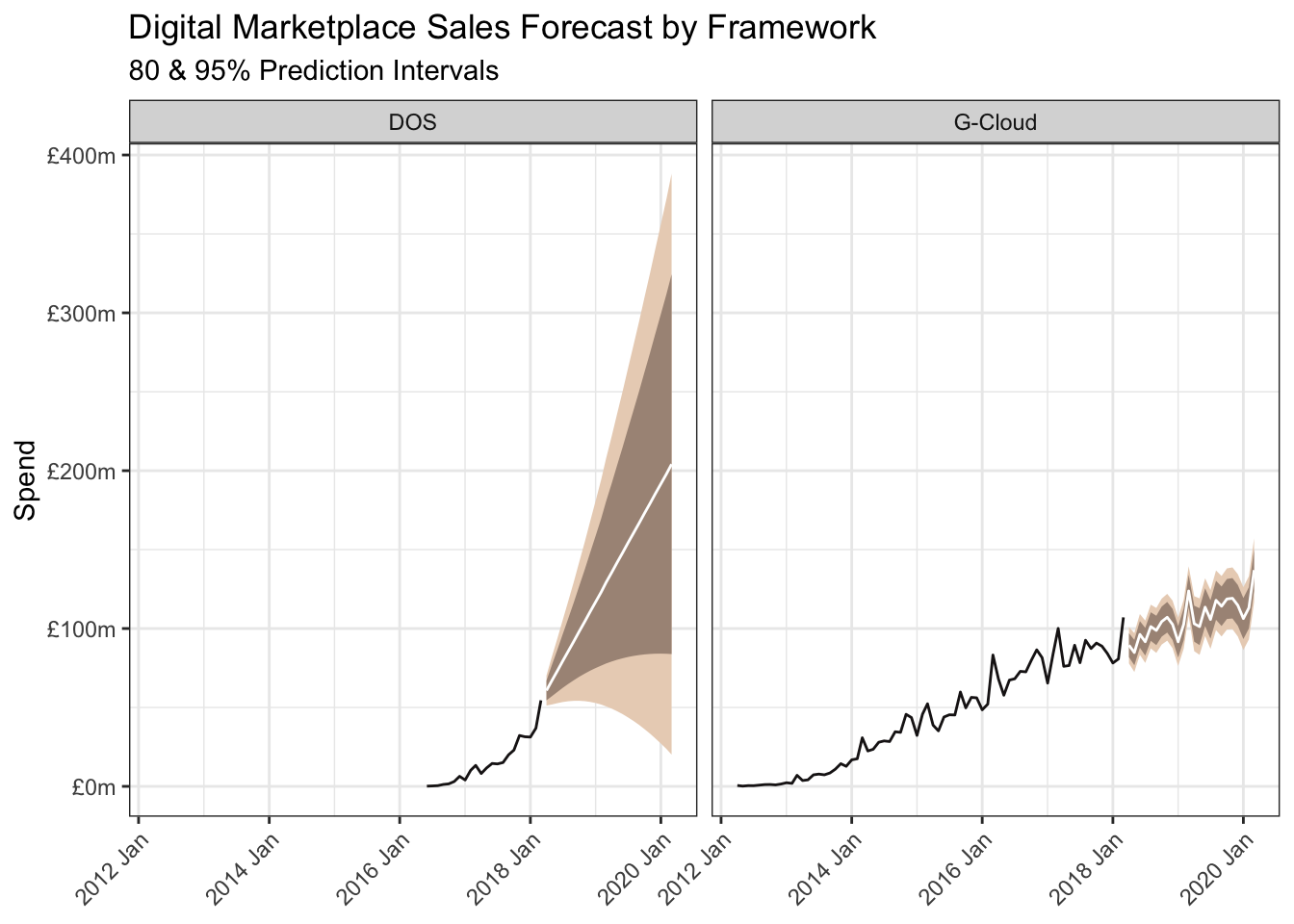The G-Cloud framework compromises three lots: Cloud Hosting, Cloud Software and Cloud Support.

I previously combined `auto.arima` (from the forecast package) with functions from the sweep package, to create multiple forecasts in one shot. tsibble coupled fabletools handle this with the `key` set to the `lot` variable.

An alternative option is hierarchical time-series forecasting which models bottom-up, top-down or middle-out, and ensures the sum of the forecasts at the lower level sum to the top-level forecast. This approach has pros and cons and is not considered here.

``````gcloud_ts <- both |>
filter(framework == "G-Cloud") |>
summarise(spend = sum(spend), .by = c(date, lot)) |>
as_tsibble(key = lot, index = date)

gc_ts <- gcloud_ts |>
model(ARIMA = ARIMA(spend, stepwise = TRUE, approximation = FALSE))

gc_ts |>
glance() |>
select(-ar_roots, -ma_roots)``````
lot .model sigma2 log_lik AIC AICc BIC
Cloud Hosting ARIMA 1.347179 -109.3173 224.6346 224.9982 231.3801
Cloud Software ARIMA 2.275664 -107.5551 221.1102 221.5465 227.3428
Cloud Support ARIMA 18.606529 -212.6556 435.3112 436.2342 446.6246
``gc_ts |> tidy()``
lot .model term estimate std.error statistic p.value
Cloud Hosting ARIMA ma1 -0.8269314 0.0765747 -10.799011 0.0000000
Cloud Hosting ARIMA constant 0.1713346 0.0258894 6.617935 0.0000000
Cloud Software ARIMA ma1 -0.9981867 0.1304426 -7.652304 0.0000000
Cloud Software ARIMA ma2 0.2242994 0.1224834 1.831264 0.0721116
Cloud Support ARIMA ma1 -0.7044948 0.0743797 -9.471597 0.0000000
Cloud Support ARIMA sma1 0.3878660 0.1458579 2.659205 0.0096735
Cloud Support ARIMA sma2 0.7866476 0.4284425 1.836064 0.0705352
Cloud Support ARIMA constant 0.7601155 0.2889763 2.630373 0.0104520
``````fcgc_ts <- gc_ts |>
forecast(h = "2 years") |>
mutate(`95%` = hilo(spend, 95), `80%` = hilo(spend, 80)) |>
unpack_hilo(c("95%", "80%")) |>
rename(fc_spend = spend) |>
bind_rows(gcloud_ts)

fcgc_ts |>
ggplot(aes(date, fill = lot)) +
geom_line(aes(y = spend), colour = cols) +
geom_ribbon(aes(ymin = `95%_lower`, ymax = `95%_upper`),
fill = cols, colour = NA
) +
geom_ribbon(aes(ymin = `80%_lower`, ymax = `80%_upper`),
fill = cols, colour = NA
) +
geom_line(aes(y = .mean), colour = "white") +
scale_y_continuous(labels = label_dollar(prefix = "£", suffix = "m")) +
facet_wrap(~lot) +
labs(
title = "G-Cloud Sales Forecast by Lot",
x = NULL, y = "Spend",
subtitle = "80 & 95% Prediction Intervals"
) +
theme(
legend.position = "none",
axis.text.x = element_text(angle = 45, hjust = 1)
)``````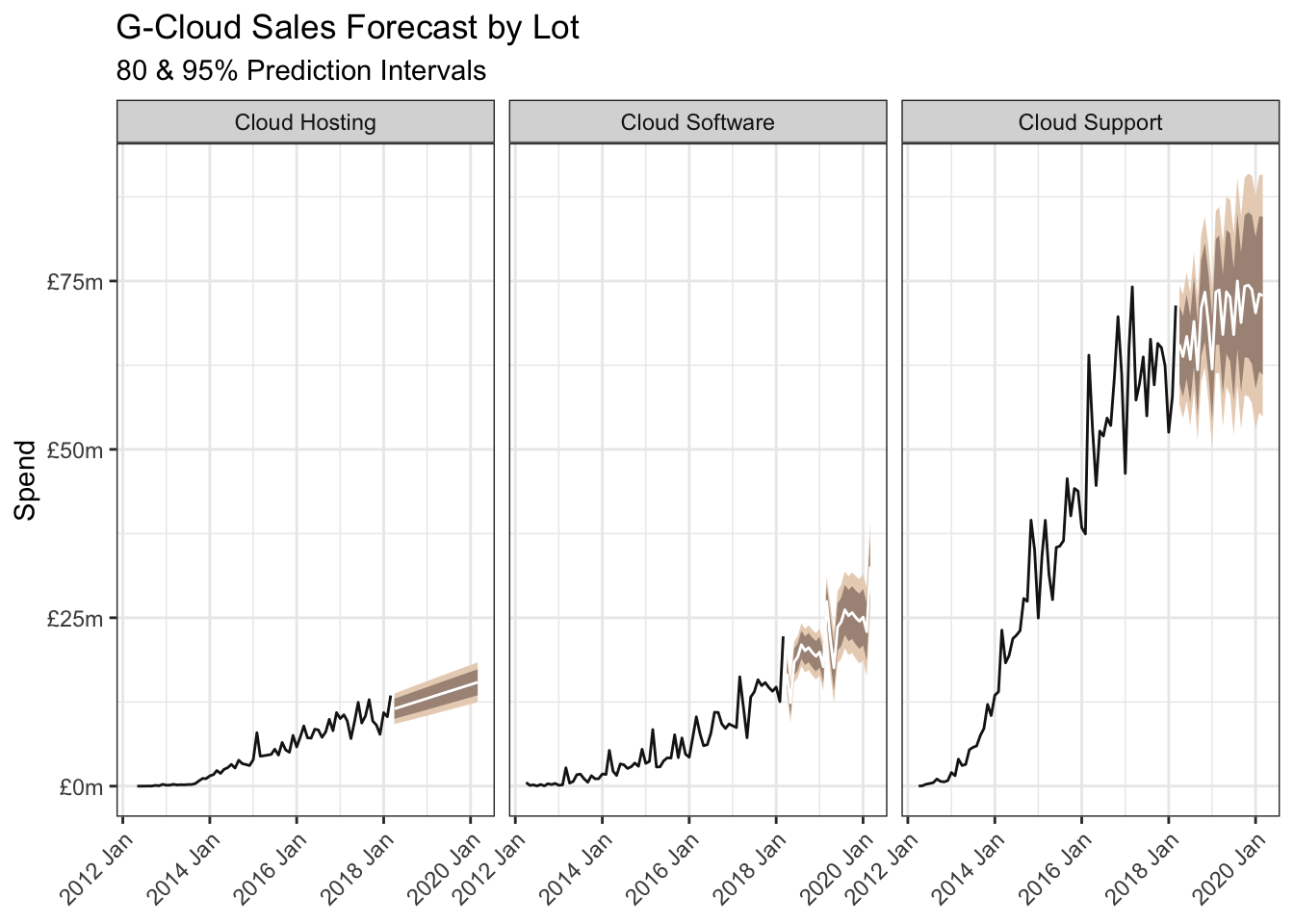So ravens are not yet ready for forecasting with R. But then neither are 4-year-olds, are they?

## R Toolbox

Summarising below the packages and functions used in this post enables me to separately create a toolbox visualisation summarising the usage of packages and functions across all posts.

``used_here()``
Package Function
base c, is.na, library, print, sum
conflicted conflict_prefer_all, conflict_scout
dplyr bind_rows, filter, if_else, mutate, recode, rename, select, summarise
fable ARIMA
fabletools components, forecast, glance, hilo, model, tidy, unpack_hilo
feasts STL
ggplot2 aes, autoplot, element_text, facet_wrap, geom_line, geom_ribbon, ggplot, labs, scale_colour_manual, scale_y_continuous, theme, theme_bw, theme_set
purrr map, walk